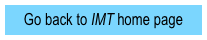Tutorials on damped and/or forced oscillations

Damped harmonic motion:  Motion graphsPretest (.pdf)

Tutorial (.pdf)

Homework (.pdf)

Exam questions (.docx)

Instructor notes (.pdf)

All materials (~1MB .zip,

.docx and .pdf)

Tutorial title

Emphasis (what do students do?)

Apply Newton’s laws to account for motion of underdamped oscillators, including the effect of damping on oscillation frequency

Connect mathematical and physical descriptions of underdamped oscillators

Damped harmonic motion:  Energy loss and the quality factorContact information for co-PIs

Department of Physics

Grand Valley State University

Allendale, MI 49401

Tel.:  616-331-2524

FAX:  616-331-3740

Email:  ambroseb@gvsu.edu

Michael C. Wittmann

Department of Physics and Astronomy

5709 Bennett Hall

University of Maine

Orono, ME  04401-5709

Tel.:  207-581-1237

FAX:  207-581-3410

Email:  wittmann@umit.maine.edu

Perform guided derivation of quality factor, expressed in terms of damping constant
and frequency

Connect motion graphs to mathematical descriptions of underdamped oscillatorsGraphical interpretation of differential equations

Pretest (.pdf)

Tutorial (.pdf)

Homework (.pdf)

All materials (~1MB .zip,

.docx and .pdf)

Analyze qualitatively the differential equations that govern motion of simple harmonic and damped oscillators

Graphical analysis of solutions, focusing on:  axis crossings (x = 0), turning points (v = 0), and inflection points (a = 0)

Pretest (.pdf)

Tutorial (.pdf)

Homework (.pdf)

Exam questions (.docx)

Instructor notes (.pdf)

All materials (~1MB .zip,

.docx and .pdf)Forced harmonic motion

Apply work and energy concepts to understand meanings of steady-state motion and resonance

Explore effect of the phase difference between driving force and position on work delivered by driver

Pretest (.pdf)

Tutorial (.pdf)

Homework (.pdf)

Exam questions (.docx)

Instructor notes (.pdf)

All materials (~1.3MB .zip,

.docx and .pdf)

Sponsored in part by NSF grants DUE-0441426 and DUE-0442388Intermediate Mechanics Tutorials are modeled after:

1. Tutorials in Introductory Physics, L.C. McDermott P.S. Shaffer, and the Physics Education Group at the University of Washington (Prentice Hall, 2002)

1. Activity-Based Tutorials, M.C. Wittmann, R.N. Steinberg, E.F. Redish, and the University of Maryland Physics Education Research Group (Wiley, 2004 and 2005)